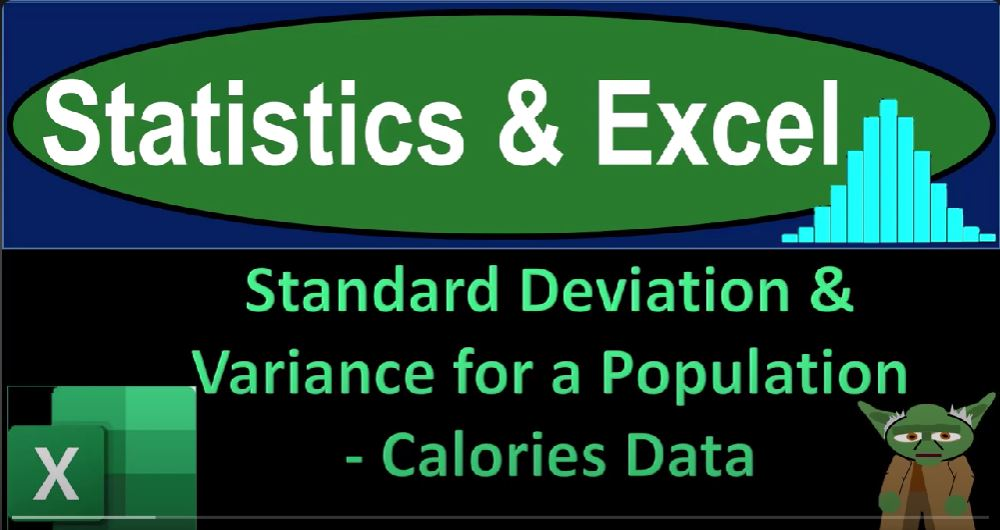## Standard Deviation & Variance for a Population – Calories Data 1458 Statistics & Excel

In this blog post, we will explore statistical calculations for a population’s calorie data using Microsoft Excel. We will focus on calculating standard deviation and variance for the population.

### Preparing the Excel Sheet

1. Open Excel: If you don’t have access to the specific workbook mentioned in the text, you can start by opening a blank Excel worksheet.
2. Formatting the Cells: Format the entire worksheet for better readability. Right-click on the triangle in the upper left corner to select all cells and format them as follows:
• Change the number format to “Currency.”
• Format negative numbers in red and bracketed.
• Remove dollar signs and decimals.
3. Bold Text: Bold all the text in the sheet for clarity.
4. Formatting the Date Field: If your data includes dates, format the date column (Column A) to display short dates. This makes it easier to work with date data.

### Data Preparation

1. Insert a Table: Place your cursor within the data, then go to the “Insert” tab, and select “Table.” This step makes it easier to manipulate and analyze your data.
2. Sort Data: You can sort your data by date or calorie count, depending on your analysis needs.

### Basic Calculations

1. Calculate Basic Statistics: Calculate the following statistics for your data:
• Mean (Average)
• Minimum (Min)
• First Quartile (Q1)
• Median (Q2)
• Third Quartile (Q3)
• Maximum (Max)
2. Population Standard Deviation and Variance: Calculate the population standard deviation and variance using the following formulas:
• Population Variance: `=VAR.P(data)`
• Population Standard Deviation: `=STDEV.P(data)`

### Manual Calculation of Variance and Standard Deviation

1. Manual Calculation: To understand how variance and standard deviation are calculated, you can manually compute them using the following steps:
• Copy your data to a new location (e.g., Column R).
• Calculate the mean (average) of your data.
• Calculate the squared difference of each data point from the mean.
• Sum up the squared differences.
• Divide the sum by the number of data points (population size) to get the population variance.
• Take the square root of the variance to get the population standard deviation.

### Symbolic Representation

1. Symbolic Representation: Use Greek letters to represent statistical symbols like sigma (σ) for standard deviation and sigma squared (σ²) for variance.
2. Formatting for Symbols: To insert Greek symbols, go to the “Insert” tab, select “Symbol,” choose the desired symbol, and format it accordingly (e.g., subscript for squared notation).

### Final Touches

1. Formatting: Add some decimals to your standard deviation and variance values to improve precision.
2. Highlighting: Consider using different colors or borders to highlight key results.

By following these steps and using Excel’s built-in functions, you can effectively calculate and understand the standard deviation and variance for a population’s calorie data. This analysis can provide insights into the data’s variability and distribution, aiding in further statistical exploration and decision-making.